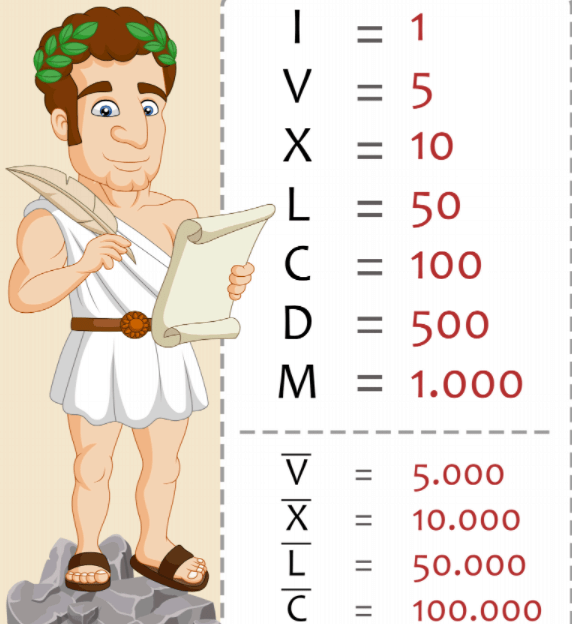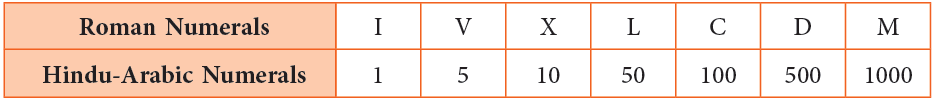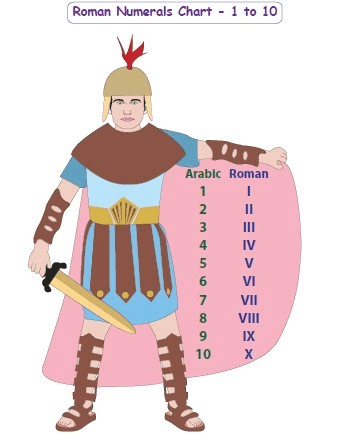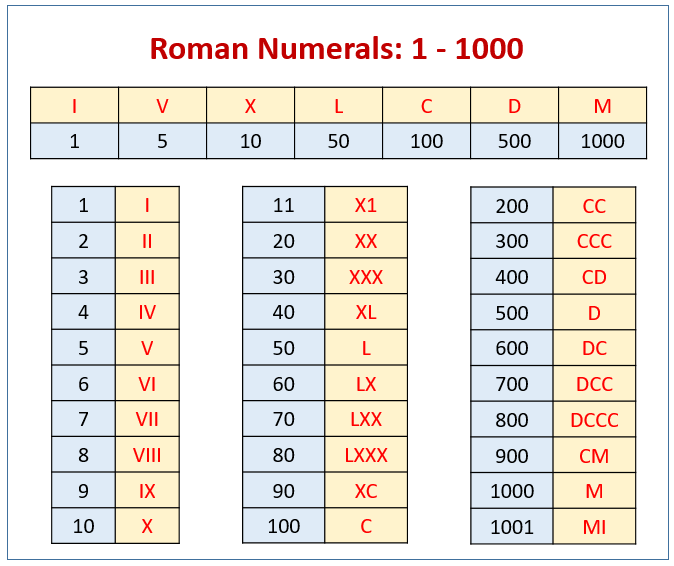# Chapter Notes: Roman Numerals Notes | Study Mathematics for Class 4 - Class 4

## Class 4: Chapter Notes: Roman Numerals Notes | Study Mathematics for Class 4 - Class 4

The document Chapter Notes: Roman Numerals Notes | Study Mathematics for Class 4 - Class 4 is a part of the Class 4 Course Mathematics for Class 4.
All you need of Class 4 at this link: Class 4

What are Roman Numerals?

Roman Numerals was a system that was the way that the Roman's would write different numbers. Instead of using numbers.

• The Hindu-Arabic numeration system uses 10 digits 0, 1, 2, 3, 4, 5, 6, 7, 8, and 9 to write any other numeral.
• The Romans had seven basic symbols to write any number. These seven symbols are I, V, X, L, C, D, and M.Basic Roman NumeralsThe Roman numerals with their corresponding Hindu-Arabic numerals are as follows:• These basic Roman symbols are combined in a specific manner to form other Roman numerals.
• Roman numerals are used in some analogue clocks and watches, to name the classrooms in the schools, for the copyright date on films programmes, etc.One to Twelve Roman Numbers written on a watch.

Try yourself:Which of the following options is the Hindu-Arabic form for the Roman numeral XXXIV?

Rules for Forming Roman Numerals

The following rules are used to write the numbers in the Roman system.

Rule Number 1

When a Roman numeral is repeated more than once, we add its value each time to get the number.

Examples:

• II = 1 + 1 = 2,
• XX = 10 + 10 = 20,
• XXX = 10 + 10 + 10 = 30

Edurev Tips:

• The same symbol is not repeated more than 3 times together.
• The symbols V, L and D are never repeated.
• There is no symbol for zero in the Roman system.
• Symbols I, X and V are used to write Roman numerals up to 39.Try yourself:Which of the following options is not written correctly according to the Roman numerals rules?

Rule Number 2

When a smaller Roman numeral is written to the right of a greater Roman numeral, the value of the greater numeral is added to the smaller numeral.

Examples:

• VI = 5 + 1 = 6,
• XII = 10 + 1 + 1 = 12,
• XXXVI = 10 + 10 + 10 + 5 + 1 = 36Rule Number 3

When a smaller Roman numeral is written to the left of a greater Roman numeral, it is always subtracted from the value of the greater numeral.

Examples:

• IV = 5 – 1 = 4,
• IX = 10 – 1 = 9

Edurev Tips:

• Symbol I  can be subtracted only once.
• The symbols V, L and D are never subtracted.

Rule Number 4

When a smaller Roman numeral is placed between two greater Roman numerals, then its value is subtracted from the value of the greater numeral on its right.

Examples:

• XIX = 10 + (10 – 1) = 10 + 9 = 19
• XXIV = 10 + 10 + (5 – 1) = 20 + 4 = 24

Example 1: Write the following in Hindu-Arabic numerals.
(a) XII
(b) VII
(c) XV
(d) XIV

(a) XII = 10 + 1 + 1 = 12
(b) VII = 5 + 1 + 1 = 7
(c) XV = 10 + 5 = 15
(d) XIV = 10 + (5 – 1) = 14

Example 2: Write the following in Roman numerals.
(a) 37
(b) 16
(c) 28
(d) 34

(a) 37 = 30 + 7 = X + X + X + V + I + I = XXXVII
(b) 16 = 10 + 6 = X + V + I = XVI
(c) 28 = 20 + 8 = X + X + V + I + I + I = XXVIII
(d) 34 = 30 + 4 = X + X + X + IV = XXXIV

The document Chapter Notes: Roman Numerals Notes | Study Mathematics for Class 4 - Class 4 is a part of the Class 4 Course Mathematics for Class 4.
All you need of Class 4 at this link: Class 4Use Code STAYHOME200 and get INR 200 additional OFF

## Mathematics for Class 4

15 videos|68 docs|32 tests

Track your progress, build streaks, highlight & save important lessons and more!

,

,

,

,

,

,

,

,

,

,

,

,

,

,

,

,

,

,

,

,

,

;